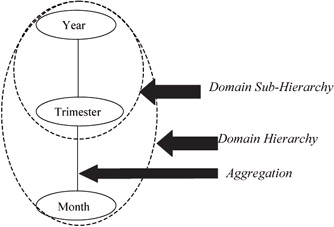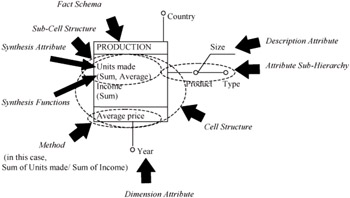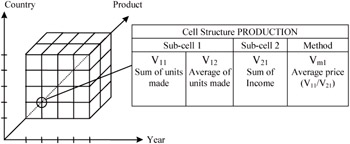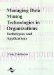# Multidimensional Conceptual Model

 < Day Day Up >

IDEA (Sánchez, 2001) is the multi dimensional conceptual data model used for conceptual modeling of multidimensional data warehouses. As every data model, it consists of a static part, which deals with data structures, and a dynamic part, which deals with data manipulation.

The elements that define the storage structures are described in the static part of IDEA. Since IDEA is an analytical, multidimensional data model, the main purpose is to serve as a basis for data analysis. Next, the static part of IDEA is briefly and informally described.

IDEA establishes a classification of nonexclusive kinds of domains:

• Dimension Domains: a dimension domain is used to represent dimension values. Dimension attributes are defined on a dimension domain. There are two kinds of dimension domains:

• OID Domains: this kind of domain is used to represent objects (products, employees, ...). By means of this domain, operational original data can be included in a multidimensional schema, and a link between elementary and multidimensional databases can be established.

• Category Domain: values of category domains are qualitative, usually extensionally defined. Not only dimension attributes can be of category domain, also description attributes (attributes used to describe a dimension attribute) can be defined on a category domain.

• Synthesis Domains: a synthesis domain is used to represent synthesis attributes of fact schemas (see below). There are two kinds of synthesis domains:

• Quantity Domains: are the most common domains used in synthesis attributes. They are intensionally defined, and mathematical operations can be applied on them.

• Boolean Domains: Boolean domains are used to indicate existence or not of information for a given subcell of the multidimensional space.

• Description Domains: a description domain is used to represent description attributes, which represent complementary information about dimension attributes. Description domains can be the already defined category or quantity domains.

Aggregations, hierarchies and sub-hierarchies can be defined on domains. An aggregation consists of an aggregation function and two dimension domains, being one of them the origin and the other the destination. The aggregation function is a mathematical function that makes a correspondence between both domains. A hierarchy is a set of domain aggregations. It is graphically represented by a graph in which each node represents a dimension domain, and each arc represents an aggregation function. A sub-hierarchy is a set of domain aggregations contained in a hierarchy. That is, a domain sub-hierarchy is a subgraph of a hierarchy graph. On a domain sub-hierarchy can be defined an attribute sub-hierarchy, which can be the basis of a dimension, as we will see later. Figure 1 shows an example of domain hierarchy.Figure 1: Example of domain aggregation, hierarchy and sub-hierarchy

A fact schema describes a n-dimensional space related to a fact of interest for analytical processing. A fact schema consists of a set of dimensions, the dimension attributes associated to each dimension, a cell structure and, optionally, a predicate.

A dimension is defined on a dimension domain, and is defined by a dimension attribute that could be (or not) the root of an attribute sub-hierarchy.

Every cell structure is composed of substructures named subcell structures and methods applied to them. Each subcell structure consists of one synthesis attribute (defined on a synthesis domain), and a set of synthesis functions that represents how operational data have been processed to obtain summarized data (for example, sum, frequency, average, maximum, minimum,...). Synthesis functions and methods can return more than one value.

Figure 2 shows an example (graphical notation is based on (Golfarelli & Rizzi, 1999)) that represents sum and average of units made, sum of income and average price along time (year), country and product.Figure 2: Graphical representation of a fact schema

Until now, we have just described the static part of the IDEA conceptual model, that is, its structural part at intensional level. The extensional level of this model, that is, the cube, concerns to content of the n-dimensional space defined on the fact schema in a certain moment (n is the number of dimensions).

For each subcell: if the synthesis attribute is defined on a Boolean domain, then it must not have a synthesis function, so the content of the subcell should be "True" or "False." If the synthesis attribute is defined on a quantity domain and it does not have a synthesis function, the subcell should contain only one data, coming from operational original source, so no synthesis has been applied on them. If there are synthesis functions, each subcell should contain one value for each function (or more than one in the case of functions that return more than one value, such as maximum(n), minimum(n), and so on).

Figure 3 shows a cube of the example of Figure 2. A cell should be identified by its dimensions, and should contain values.Figure 3: Cube corresponding to Figure 2

 < Day Day Up >Managing Data Mining Technologies in Organizations: Techniques and Applications
ISBN: 1591400570
EAN: 2147483647
Year: 2003
Pages: 174